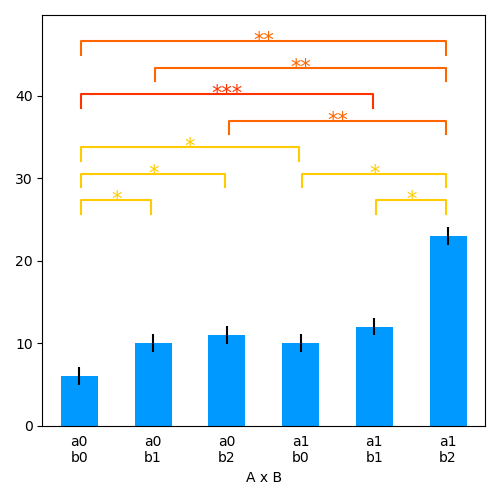# Repeated measures ANOVA

Repeated measures analysis of variance for univariate data.

Based on 1.

```# Author: Christian Brodbeck <christianbrodbeck@nyu.edu>
from eelbrain import *

y = Var([7,  3,  6,  6,  5,  8,  6,  7,
7, 11,  9, 11, 10, 10, 11, 11,
8, 14, 10, 11, 12, 10, 11, 12],
name='y')
a = Factor('abc', repeat=8, name='A')
```

Fixed effects ANOVA (independent measures, 1 p. 24):

```test.ANOVA(y, a, title="Independent Measures")
```

Repeated measures ANOVA (1 p. 72): `subject` is defined as random effect and entered for model construction as completely crossed factor

```subject = Factor(range(8), tile=3, name='subject', random=True)
test.ANOVA(y, a * subject, title="Repeated Measures")
```

## Two-way repeated measures ANOVA

```y = Var([ 7,  3,  6,  6,  5,  8,  6,  7,
7, 11,  9, 11, 10, 10, 11, 11,
8, 14, 10, 11, 12, 10, 11, 12,
16,  7, 11,  9, 10, 11,  8,  8,
16, 10, 13, 10, 10, 14, 11, 12,
24, 29, 10, 22, 25, 28, 22, 24])
a = Factor(['a0', 'a1'], repeat=3 * 8, name='A')
b = Factor(['b0', 'b1', 'b2'], tile=2, repeat=8, name='B')
subject = Factor(range(8), tile=6, name='subject', random=True)

test.ANOVA(y, a * b * subject, title="Repeated Measure:")
```

Bar-plot with within-subject error bars and pairwise tests

```p = plot.Barplot(y, a % b, match=subject)
```